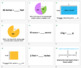# GRADE 4 Math MD Bingo - Measurement and DataSubject
Resource Type
File Type
Zip (8 MB|45 pages)
Standards
\$2.00
List Price:
\$2.50
You Save:
\$0.50
\$2.00
List Price:
\$2.50
You Save:
\$0.50
• Product Description
• Standards
A fun, engaging game to review the Common Core Standards for Grade 4 Measurement and Data. Including questions on measurement conversion, line plots and volume.

- 30 Printable Bingo Cards
- 28 Bingo Questions - in the form of PowerPoint slides so you can show the questions up in front of the class for them to solve - if they have the answer on their bingo card they cross it off. Featuring questions covering most of the MD standards, however some of the more potentially time consuming types of question are not included (eg. additive volumes) as the idea is that it's a fairly fast-pace game.
- Instructions

Recognize angle measure as additive. When an angle is decomposed into non-overlapping parts, the angle measure of the whole is the sum of the angle measures of the parts. Solve addition and subtraction problems to find unknown angles on a diagram in real world and mathematical problems, e.g., by using an equation with a symbol for the unknown angle measure.
Measure angles in whole-number degrees using a protractor. Sketch angles of specified measure.
An angle that turns through 𝘯 one-degree angles is said to have an angle measure of 𝘯 degrees.
An angle is measured with reference to a circle with its center at the common endpoint of the rays, by considering the fraction of the circular arc between the points where the two rays intersect the circle. An angle that turns through 1/360 of a circle is called a “one-degree angle,” and can be used to measure angles.
Recognize angles as geometric shapes that are formed wherever two rays share a common endpoint, and understand concepts of angle measurement:
Total Pages
45 pages